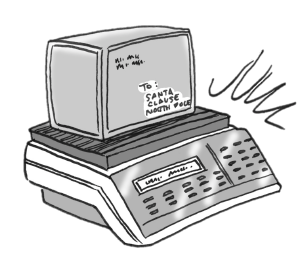### Home > CC3MN > Chapter 5 Unit 5 > Lesson CC3: 5.2.4 > Problem5-59

5-59.

Mailboxes Plus sends packages overnight for $\5$ plus $\0.25$ per ounce. United Packages charges $\2$ plus $\0.35$ per ounce. Mr. Molinari noticed that his package would cost the same to mail using either service. How much does his package weigh?Write equations for both brands.

$y=$ total cost
$x=$ weight in ounces

Mailboxes Plus: $y=0.25x+5$
United Packages: Write a similar equation.

Set the equations equal to each other to solve for $x$.

Complete the tables in the eTool below to graph the results.
Click the link at right for the full version of the eTool: CC3 5-59 HW eTool